# 10.5 Polar form of complex numbers  (Page 4/8)

 Page 4 / 8

## Finding the n Th root of a complex number

Evaluate the cube roots of $\text{\hspace{0.17em}}z=8\left(\mathrm{cos}\left(\frac{2\pi }{3}\right)+i\mathrm{sin}\left(\frac{2\pi }{3}\right)\right).$

We have

$\begin{array}{l}{z}^{\frac{1}{3}}={8}^{\frac{1}{3}}\left[\mathrm{cos}\left(\frac{\frac{2\pi }{3}}{3}+\frac{2k\pi }{3}\right)+i\mathrm{sin}\left(\frac{\frac{2\pi }{3}}{3}+\frac{2k\pi }{3}\right)\right]\hfill \\ {z}^{\frac{1}{3}}=2\left[\mathrm{cos}\left(\frac{2\pi }{9}+\frac{2k\pi }{3}\right)+i\mathrm{sin}\left(\frac{2\pi }{9}+\frac{2k\pi }{3}\right)\right]\hfill \end{array}$

There will be three roots: $\text{\hspace{0.17em}}k=0,\text{\hspace{0.17em}}\text{\hspace{0.17em}}1,\text{\hspace{0.17em}}\text{\hspace{0.17em}}2.\text{\hspace{0.17em}}$ When $\text{\hspace{0.17em}}k=0,\text{\hspace{0.17em}}$ we have

${z}^{\frac{1}{3}}=2\left(\mathrm{cos}\left(\frac{2\pi }{9}\right)+i\mathrm{sin}\left(\frac{2\pi }{9}\right)\right)$

When $\text{\hspace{0.17em}}k=1,\text{\hspace{0.17em}}$ we have

When $\text{\hspace{0.17em}}k=2,\text{\hspace{0.17em}}$ we have

Remember to find the common denominator to simplify fractions in situations like this one. For $\text{\hspace{0.17em}}k=1,\text{\hspace{0.17em}}$ the angle simplification is

$\begin{array}{l}\frac{\frac{2\pi }{3}}{3}+\frac{2\left(1\right)\pi }{3}=\frac{2\pi }{3}\left(\frac{1}{3}\right)+\frac{2\left(1\right)\pi }{3}\left(\frac{3}{3}\right)\hfill \\ \text{\hspace{0.17em}}\text{\hspace{0.17em}}\text{\hspace{0.17em}}\text{\hspace{0.17em}}\text{\hspace{0.17em}}\text{\hspace{0.17em}}\text{\hspace{0.17em}}\text{\hspace{0.17em}}\text{\hspace{0.17em}}\text{\hspace{0.17em}}\text{\hspace{0.17em}}\text{\hspace{0.17em}}\text{\hspace{0.17em}}\text{\hspace{0.17em}}\text{\hspace{0.17em}}\text{\hspace{0.17em}}\text{\hspace{0.17em}}\text{\hspace{0.17em}}\text{\hspace{0.17em}}\text{\hspace{0.17em}}\text{\hspace{0.17em}}\text{\hspace{0.17em}}\text{\hspace{0.17em}}\text{\hspace{0.17em}}\text{\hspace{0.17em}}\text{\hspace{0.17em}}=\frac{2\pi }{9}+\frac{6\pi }{9}\hfill \\ \text{\hspace{0.17em}}\text{\hspace{0.17em}}\text{\hspace{0.17em}}\text{\hspace{0.17em}}\text{\hspace{0.17em}}\text{\hspace{0.17em}}\text{\hspace{0.17em}}\text{\hspace{0.17em}}\text{\hspace{0.17em}}\text{\hspace{0.17em}}\text{\hspace{0.17em}}\text{\hspace{0.17em}}\text{\hspace{0.17em}}\text{\hspace{0.17em}}\text{\hspace{0.17em}}\text{\hspace{0.17em}}\text{\hspace{0.17em}}\text{\hspace{0.17em}}\text{\hspace{0.17em}}\text{\hspace{0.17em}}\text{\hspace{0.17em}}\text{\hspace{0.17em}}\text{\hspace{0.17em}}\text{\hspace{0.17em}}\text{\hspace{0.17em}}\text{\hspace{0.17em}}=\frac{8\pi }{9}\hfill \end{array}$

Find the four fourth roots of $\text{\hspace{0.17em}}16\left(\mathrm{cos}\left(120°\right)+i\mathrm{sin}\left(120°\right)\right).$

${z}_{0}=2\left(\mathrm{cos}\left(30°\right)+i\mathrm{sin}\left(30°\right)\right)$

${z}_{1}=2\left(\mathrm{cos}\left(120°\right)+i\mathrm{sin}\left(120°\right)\right)$

${z}_{2}=2\left(\mathrm{cos}\left(210°\right)+i\mathrm{sin}\left(210°\right)\right)$

${z}_{3}=2\left(\mathrm{cos}\left(300°\right)+i\mathrm{sin}\left(300°\right)\right)$

Access these online resources for additional instruction and practice with polar forms of complex numbers.

## Key concepts

• Complex numbers in the form $\text{\hspace{0.17em}}a+bi\text{\hspace{0.17em}}$ are plotted in the complex plane similar to the way rectangular coordinates are plotted in the rectangular plane. Label the x- axis as the real axis and the y- axis as the imaginary axis. See [link] .
• The absolute value of a complex number is the same as its magnitude. It is the distance from the origin to the point: $\text{\hspace{0.17em}}|z|=\sqrt{{a}^{2}+{b}^{2}}.\text{\hspace{0.17em}}$ See [link] and [link] .
• To write complex numbers in polar form, we use the formulas $\text{\hspace{0.17em}}x=r\mathrm{cos}\text{\hspace{0.17em}}\theta ,y=r\mathrm{sin}\text{\hspace{0.17em}}\theta ,\text{\hspace{0.17em}}$ and $\text{\hspace{0.17em}}r=\sqrt{{x}^{2}+{y}^{2}}.\text{\hspace{0.17em}}$ Then, $\text{\hspace{0.17em}}z=r\left(\mathrm{cos}\text{\hspace{0.17em}}\theta +i\mathrm{sin}\text{\hspace{0.17em}}\theta \right).\text{\hspace{0.17em}}$ See [link] and [link] .
• To convert from polar form to rectangular form, first evaluate the trigonometric functions. Then, multiply through by $\text{\hspace{0.17em}}r.\text{\hspace{0.17em}}$ See [link] and [link] .
• To find the product of two complex numbers, multiply the two moduli and add the two angles. Evaluate the trigonometric functions, and multiply using the distributive property. See [link] .
• To find the quotient of two complex numbers in polar form, find the quotient of the two moduli and the difference of the two angles. See [link] .
• To find the power of a complex number $\text{\hspace{0.17em}}{z}^{n},\text{\hspace{0.17em}}$ raise $\text{\hspace{0.17em}}r\text{\hspace{0.17em}}$ to the power $\text{\hspace{0.17em}}n,$ and multiply $\text{\hspace{0.17em}}\theta \text{\hspace{0.17em}}$ by $\text{\hspace{0.17em}}n.\text{\hspace{0.17em}}$ See [link] .
• Finding the roots of a complex number is the same as raising a complex number to a power, but using a rational exponent. See [link] .

## Verbal

A complex number is $\text{\hspace{0.17em}}a+bi.\text{\hspace{0.17em}}$ Explain each part.

a is the real part, b is the imaginary part, and $\text{\hspace{0.17em}}i=\sqrt{-1}$

What does the absolute value of a complex number represent?

How is a complex number converted to polar form?

Polar form converts the real and imaginary part of the complex number in polar form using $\text{\hspace{0.17em}}x=r\mathrm{cos}\theta \text{\hspace{0.17em}}$ and $\text{\hspace{0.17em}}y=r\mathrm{sin}\theta .$

How do we find the product of two complex numbers?

What is De Moivre’s Theorem and what is it used for?

${z}^{n}={r}^{n}\left(\mathrm{cos}\left(n\theta \right)+i\mathrm{sin}\left(n\theta \right)\right)\text{\hspace{0.17em}}$ It is used to simplify polar form when a number has been raised to a power.

## Algebraic

For the following exercises, find the absolute value of the given complex number.

$5+\text{​}3i$

$-7+\text{​}i$

$5\sqrt{2}$

$-3-3i$

$\sqrt{2}-6i$

$\sqrt{38}$

$2i$

$2.2-3.1i$

$\sqrt{14.45}$

For the following exercises, write the complex number in polar form.

$2+2i$

$8-4i$

$4\sqrt{5}\mathrm{cis}\left(333.4°\right)$

$-\frac{1}{2}-\frac{1}{2}\text{​}i$

$\sqrt{3}+i$

$2\mathrm{cis}\left(\frac{\pi }{6}\right)$

$3i$

For the following exercises, convert the complex number from polar to rectangular form.

$z=7\mathrm{cis}\left(\frac{\pi }{6}\right)$

$\frac{7\sqrt{3}}{2}+i\frac{7}{2}$

$z=2\mathrm{cis}\left(\frac{\pi }{3}\right)$

$z=4\mathrm{cis}\left(\frac{7\pi }{6}\right)$

$-2\sqrt{3}-2i$

$z=7\mathrm{cis}\left(25°\right)$

$z=3\mathrm{cis}\left(240°\right)$

$-1.5-i\frac{3\sqrt{3}}{2}$

$z=\sqrt{2}\mathrm{cis}\left(100°\right)$

For the following exercises, find $\text{\hspace{0.17em}}{z}_{1}{z}_{2}\text{\hspace{0.17em}}$ in polar form.

$4\sqrt{3}\mathrm{cis}\left(198°\right)$

$\frac{3}{4}\mathrm{cis}\left(180°\right)$

$5\sqrt{3}\mathrm{cis}\left(\frac{17\pi }{24}\right)$

For the following exercises, find $\text{\hspace{0.17em}}\frac{{z}_{1}}{{z}_{2}}\text{\hspace{0.17em}}$ in polar form.

$7\mathrm{cis}\left(70°\right)$

$5\mathrm{cis}\left(80°\right)$

$5\mathrm{cis}\left(\frac{\pi }{3}\right)$

For the following exercises, find the powers of each complex number in polar form.

Find $\text{\hspace{0.17em}}{z}^{3}\text{\hspace{0.17em}}$ when $\text{\hspace{0.17em}}z=5\mathrm{cis}\left(45°\right).$

$125\mathrm{cis}\left(135°\right)$

Find $\text{\hspace{0.17em}}{z}^{4}\text{\hspace{0.17em}}$ when $\text{\hspace{0.17em}}z=2\mathrm{cis}\left(70°\right).$

Find $\text{\hspace{0.17em}}{z}^{2}\text{\hspace{0.17em}}$ when $\text{\hspace{0.17em}}z=3\mathrm{cis}\left(120°\right).$

$9\mathrm{cis}\left(240°\right)$

Find $\text{\hspace{0.17em}}{z}^{2}\text{\hspace{0.17em}}$ when $\text{\hspace{0.17em}}z=4\mathrm{cis}\left(\frac{\pi }{4}\right).$

Find $\text{\hspace{0.17em}}{z}^{4}\text{\hspace{0.17em}}$ when $\text{\hspace{0.17em}}z=\mathrm{cis}\left(\frac{3\pi }{16}\right).$

$\mathrm{cis}\left(\frac{3\pi }{4}\right)$

Find $\text{\hspace{0.17em}}{z}^{3}\text{\hspace{0.17em}}$ when $\text{\hspace{0.17em}}z=3\mathrm{cis}\left(\frac{5\pi }{3}\right).$

For the following exercises, evaluate each root.

Evaluate the cube root of $\text{\hspace{0.17em}}z\text{\hspace{0.17em}}$ when $\text{\hspace{0.17em}}z=27\mathrm{cis}\left(240°\right).$

$\text{\hspace{0.17em}}3\mathrm{cis}\left(80°\right),3\mathrm{cis}\left(200°\right),3\mathrm{cis}\left(320°\right)\text{\hspace{0.17em}}$

Evaluate the square root of $\text{\hspace{0.17em}}z\text{\hspace{0.17em}}$ when $\text{\hspace{0.17em}}z=16\mathrm{cis}\left(100°\right).$

Evaluate the cube root of $\text{\hspace{0.17em}}z\text{\hspace{0.17em}}$ when $\text{\hspace{0.17em}}z=32\mathrm{cis}\left(\frac{2\pi }{3}\right).$

$\text{\hspace{0.17em}}2\sqrt{4}\mathrm{cis}\left(\frac{2\pi }{9}\right),2\sqrt{4}\mathrm{cis}\left(\frac{8\pi }{9}\right),2\sqrt{4}\mathrm{cis}\left(\frac{14\pi }{9}\right)$

Evaluate the square root of $\text{\hspace{0.17em}}z\text{\hspace{0.17em}}$ when $\text{\hspace{0.17em}}z=32\text{cis}\left(\pi \right).$

Evaluate the cube root of $\text{\hspace{0.17em}}z\text{\hspace{0.17em}}$ when $\text{\hspace{0.17em}}z=8\mathrm{cis}\left(\frac{7\pi }{4}\right).$

$2\sqrt{2}\mathrm{cis}\left(\frac{7\pi }{8}\right),2\sqrt{2}\mathrm{cis}\left(\frac{15\pi }{8}\right)$

## Graphical

For the following exercises, plot the complex number in the complex plane.

$2+4i$

$-3-3i$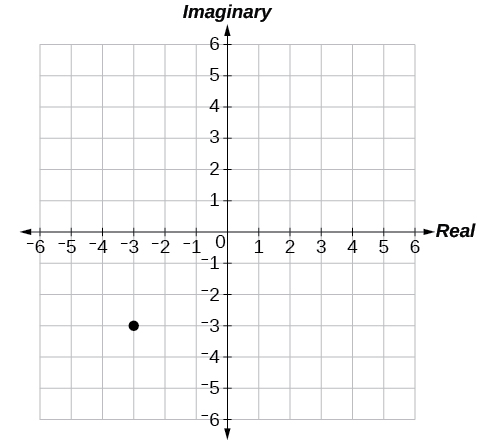$5-4i$

$-1-5i$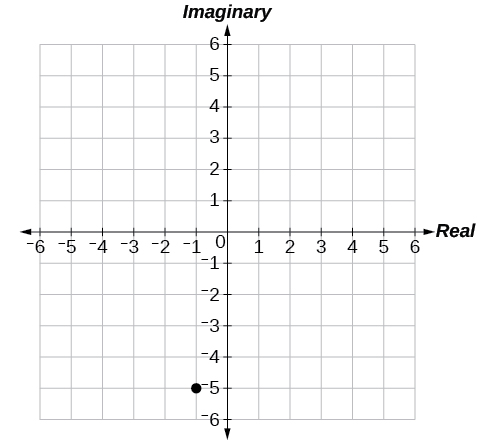$3+2i$

$2i$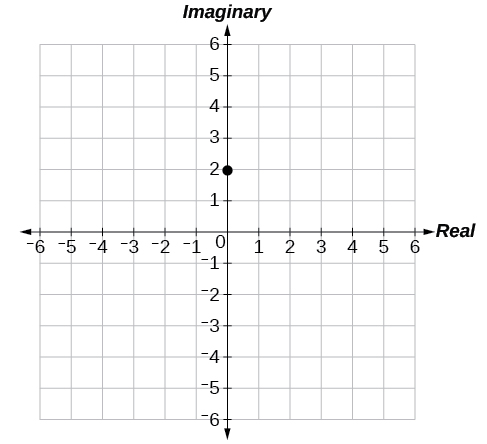$-4$

$6-2i$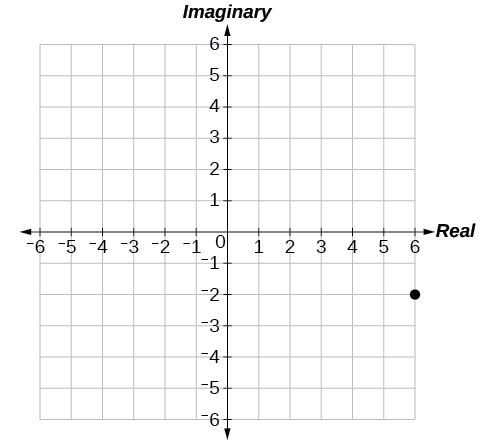$-2+i$

$1-4i$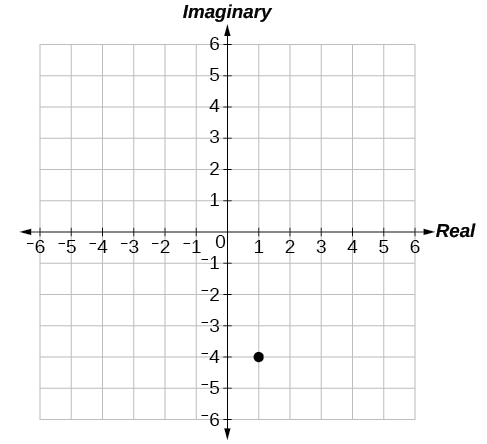## Technology

For the following exercises, find all answers rounded to the nearest hundredth.

Use the rectangular to polar feature on the graphing calculator to change $\text{\hspace{0.17em}}5+5i\text{\hspace{0.17em}}$ to polar form.

Use the rectangular to polar feature on the graphing calculator to change $\text{\hspace{0.17em}}3-2i\text{\hspace{0.17em}}$ to polar form.

$\text{\hspace{0.17em}}3.61{e}^{-0.59i}\text{\hspace{0.17em}}$

Use the rectangular to polar feature on the graphing calculator to change $-3-8i\text{\hspace{0.17em}}$ to polar form.

Use the polar to rectangular feature on the graphing calculator to change $\text{\hspace{0.17em}}4\mathrm{cis}\left(120°\right)\text{\hspace{0.17em}}$ to rectangular form.

$\text{\hspace{0.17em}}-2+3.46i\text{\hspace{0.17em}}$

Use the polar to rectangular feature on the graphing calculator to change $\text{\hspace{0.17em}}2\mathrm{cis}\left(45°\right)\text{\hspace{0.17em}}$ to rectangular form.

Use the polar to rectangular feature on the graphing calculator to change $\text{\hspace{0.17em}}5\mathrm{cis}\left(210°\right)\text{\hspace{0.17em}}$ to rectangular form.

$\text{\hspace{0.17em}}-4.33-2.50i\text{\hspace{0.17em}}$

the third and the seventh terms of a G.P are 81 and 16, find the first and fifth terms.
if a=3, b =4 and c=5 find the six trigonometric value sin
pls how do I factorize x⁴+x³-7x²-x+6=0
in a function the input value is called
how do I test for values on the number line
if a=4 b=4 then a+b=
a+b+2ab
Kin
commulative principle
a+b= 4+4=8
Mimi
If a=4 and b=4 then we add the value of a and b i.e a+b=4+4=8.
Tariq
what are examples of natural number
an equation for the line that goes through the point (-1,12) and has a slope of 2,3
3y=-9x+25
Ishaq
show that the set of natural numberdoes not from agroup with addition or multiplication butit forms aseni group with respect toaaddition as well as multiplication
x^20+x^15+x^10+x^5/x^2+1
evaluate each algebraic expression. 2x+×_2 if ×=5
if the ratio of the root of ax+bx+c =0, show that (m+1)^2 ac =b^2m
By the definition, is such that 0!=1.why?
(1+cosA+IsinA)(1+cosB+isinB)/(cos@+isin@)(cos$+isin$)
hatdog
Mark
jaks
Ryan
how we can draw three triangles of distinctly different shapes. All the angles will be cutt off each triangle and placed side by side with vertices touching

#### Get Jobilize Job Search Mobile App in your pocket Now!ByByBy OpenStaxBy Stephanie RedfernBy Stephen VoronBy Danielle StephensByBy Madison ChristianBy Ellie BanfieldBy Carly AllenBy OpenStaxBy Melinda Salzer